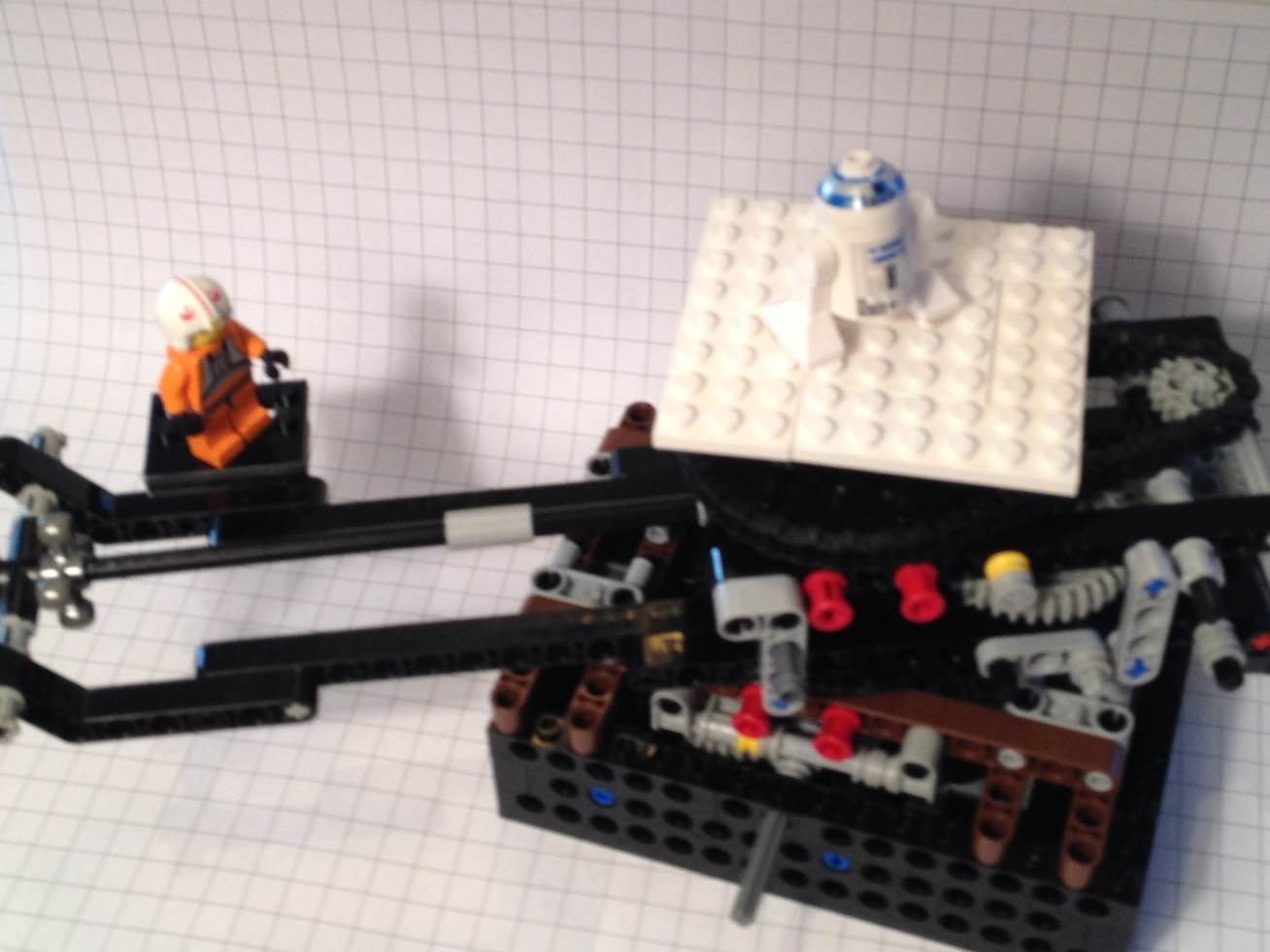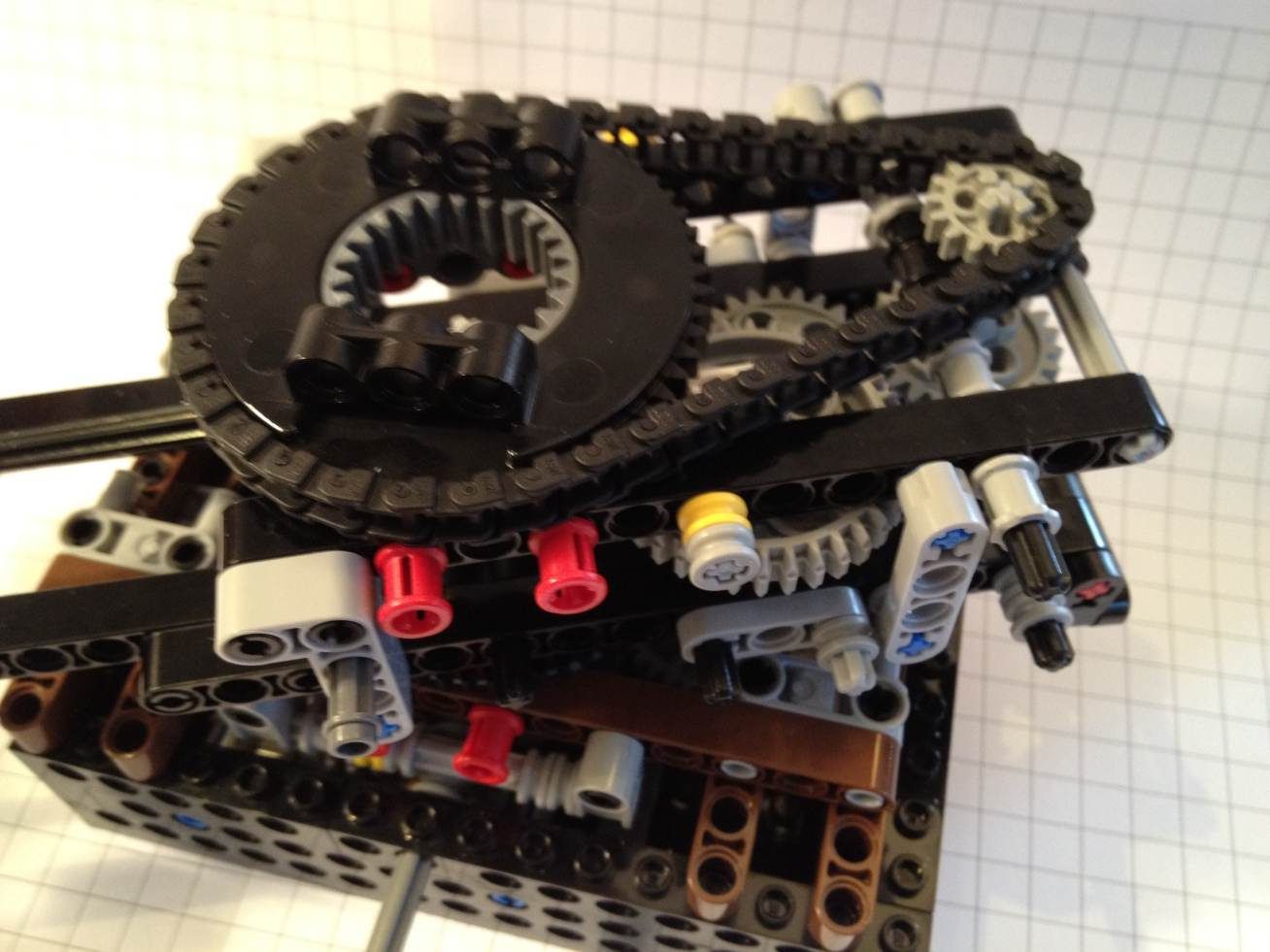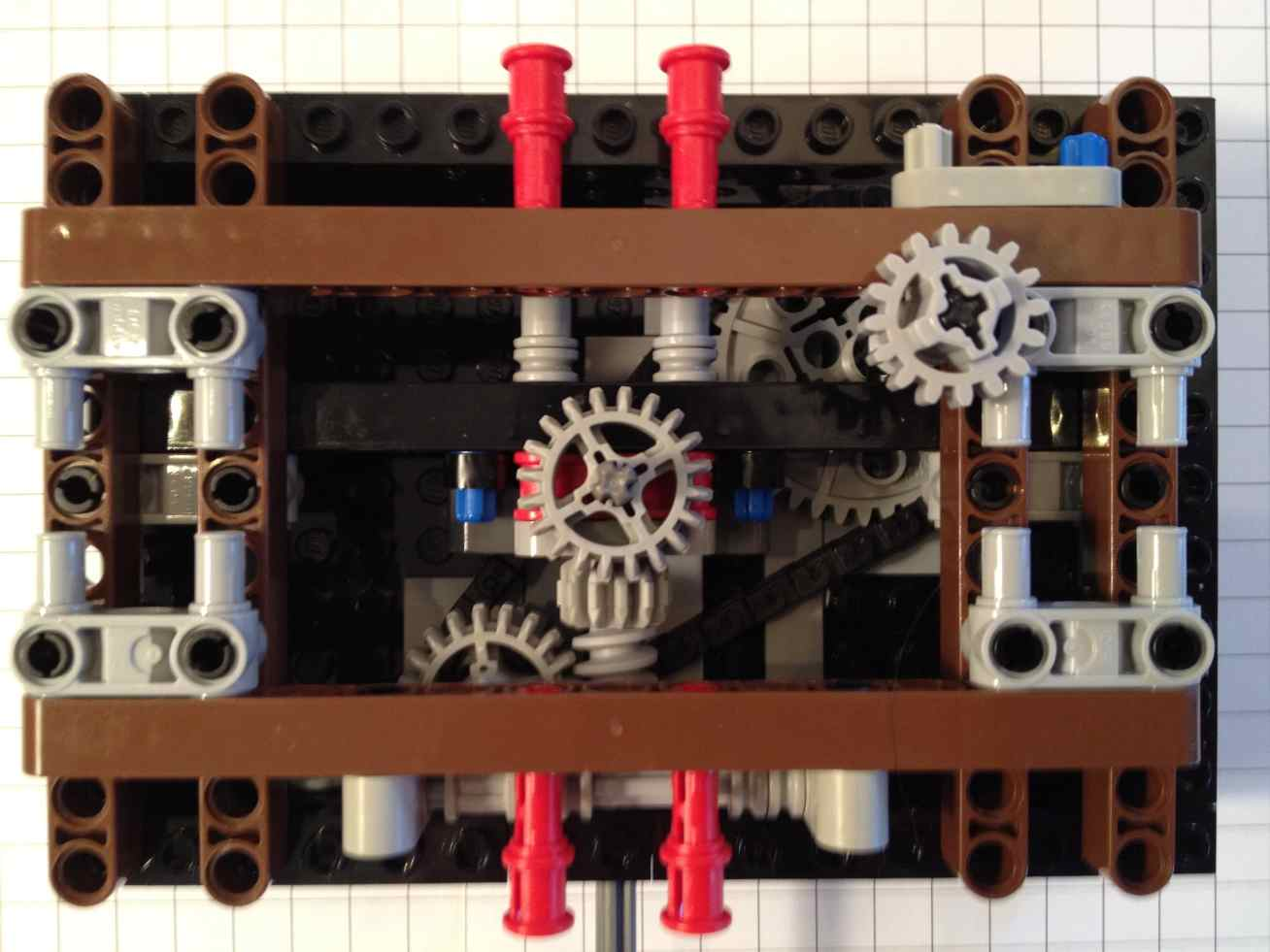mdbtxt1
mdbtxt2
Proceed to Safety

# Rotational Maths for Orrery Design

Consider the following construction:Example of rotating platforms

Here there is a base containing some gears, a rotating arm holding the "Luke Skywalker" figure (the Luke platform), and another rotating platform holding the "R2-D2" figure (the R2 platform).

From our point of view, the base is not rotating, and the other two pieces are rotating clockwise. The R2 platform is rotating faster than the Luke platform.

Throughout this discussion we will use rates of rotation, rather than periods or frequencies. To illustrate the difference, here are the rate, period and frequency for a minute hand on a clock:

minute hand on a clock
rate : 6 degrees per minute
period (time to complete 1 rotation) : 60 minutes
frequency : 1/60 of a rotation per minute (1/60 RPM)

Note that "frequency" is like "rate", but is in full turns (rotations) per unit time rather than in degrees per unit time or some other small unit of angle. They're equivalent, but since the rate uses smaller units (degrees rather than full-turns) the numbers are usually a bit more convenient and it may be easier to understand intuitively.

From the camera's point of view, we will refer to the rates of the Luke and R2 platforms as A and B respectively:

rate of Luke platform from camera point of view = Ratecamera(Luke) = A
rate of R2 platform from camera point of view = Ratecamera(R2) = B

Rotation rates can be a bit confusing if the point of view is also rotating. In this example, the gearing that rotates the R2 platform is located inside the Luke section:R2's turntable is connected by a chain to some gearsrotated 180 degrees, and showing the gearing inside

There is also a central axle which provides the mechanical power to the gearing seen above. This axle turns quite a bit faster than the two rotating platforms and the gearing in the Luke section slows it down.

rate of central axle from camera point of view = Ratecamera(axle) = C

The ratio between A and C is determined by gearing in the base:the gearing inside the base

It's a little hard to see all the gears, but the overall ratio between A and C is 105: As seen by the camera (a non-rotating reference frame), the central axle turns 105 times for each time the Luke platform turns.

Ratecamera(Luke) = 1/105 × Ratecamera(axle)
A = 105 C
C = A/105

Rotation can be perceived from different points of view. To work out how fast the R2 platform will go, we need to consider the rotation rates from Luke's point of view:

rate of R2 platform from Luke point of view = RateLuke(R2) = B-A
rate of central axle from Luke point of view = RateLuke(axle) = C-A

In my abbreviated geartrain notation, the gearing inside the Luke module consists of the following gears:

First turntable module (Luke): input = +12 -> 20+8 -> 36+16 -> 24 -> 24+16 >ooo> 56 = output (next turntable)

In English, reading from left to right, we have a 12-tooth gear meshed with a 20-tooth gear,

which is attached by an axle to a 8-tooth gear that is meshed with a 36-tooth gear,

which is attached by an axle to a 16-tooth gear that is meshed with a 24-tooth gear (an idler),

which is meshed with another 24-tooth gear,

which is attached by an axle to a 16-tooth gear that drives a chain which goes to the 56-tooth gear on the turntable of the R2 platform.

The overall ratio is:

output rate = input rate × 12/20 × 8/36 × 16/24 × 16/56
= 8/315 × input rate
= 1/39.375 × input rate
{~=} 0.025397 × input rate

This ratio of 8/315 is only observed from a reference frame in which the entire mechanism as a whole is not rotating. In other words, the ratio 8/315 is the ratio between RateLuke[R2] and RateLuke[axle]. So we have:

(RateLuke(R2) = 8/315 × RateLuke(axle)
(B-A) = 8/315 × (C-A)

We already know the ratio between A and C is 105, so we can substitute C/105 for A:

(B-C/105) = 8/315 × (C-C/105)

We want to solve for B in terms of C. To do the fractional arithmetic we need to use the least common multiple of 105 and 315, which is 33075:

B = (8/315)(C-C/105) + C/105
B = 840/33075 C - 8/33075 C + 315/33075 C
B = (840 - 8 + 315)/33075 C
B = 1147/33075 C

So now we know that for every 33075 turns of the central shaft, the R2 platform will turn 1147 times.

The prime factors of the denominator 33075 are 33×52×72. Those numbers make sense because all the gears we used have numbers that are multiples of 2, 3, 5 and 7 (the turntable itself has 56 teeth around the outer edge, 56=7×8).

However, the prime factors of 1147 are 31×37. These numbers do not appear in the gears we used, so it is a bit of a surprise that these numbers appear in the rotation rate of the R2 platform. The reason we get new prime numbers is that this mechanism essentially adds and subtracts rotation rates in addition to multiplying. When we put everything over the common denominator 33075, the separate fractions 840/33075, 8/33075 and 315/33075 are still all in terms of 2s, 3s, 5s, and 7s. But when we add and subtract the rates, the result (840-8+315 = 1147) is not a multiple of any of those small primes. A simpler example is seen in adding simple fractions:

1/3 + 1/4 + 1/5 = 47/60

The denominator is 3×4×5, but the numerator is a large prime number.

This kind of thing happens quite often in complex gearing systems, and it's part of the reason why it is easy to approximate irrational numbers (like the rates of planetary orbits) with a relatively limited set of basic gears.

LEGO® creations index

The graph paper in my newer photos is ruled at a specing of 1 LSS, which is about 7.99 mm.

This site is not affiliated with the LEGO® group of companies.

LEGO®, Duplo®, QUATRO®, DACTA®, MINDSTORMS®, Constructopedia®, Robotics Invention System® and Lego Technic®, etc. are trademarks or registered trademarks of LEGO Group. LEGO Group does not sponsor, authorize or endorse this site.

All other trademarks, service marks, and copyrights are property of their respective owners.

This page was written in the "embarrassingly readable" markup language RHTF, and was last updated on 2012 Dec 29.s.27